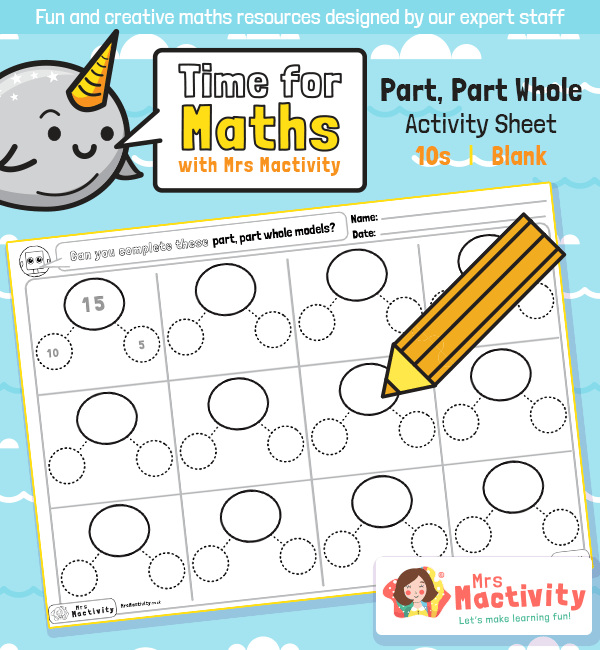# Year 1 Part Part Whole Activity SheetHelp children understand addition, subtraction and number family facts with this Year 1 Part Part Whole Activity Sheet. This covers the year 1 maths objective:

• represent and use number bonds and related subtraction facts within 20
• add and subtract one-digit and two-digit numbers to 20, including zero
• solve one-step problems that involve addition and subtraction, using concrete objects and pictorial representations, and missing number problems such as 7 = – 9.

Explore our year 1 maths resources.

Explore our maths scheme.

Explore our addition and subtraction resources.

## Recently Viewed